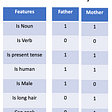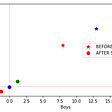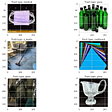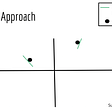# Multivariate Linear regression from Scratch Using OLS (Ordinary Least Square Estimator)

Almost all of the Machine learning algorithms focus on learning function which can describe the relationship between input (features/independent variable) and output(target Variabel/dependent variable). The form of this function depends on the algorithm used. Linear regression is one of the simplest machine learning algorithms which uses a linear function to describe the relationship between input and the target variable. A simple equation for multivariate (having more than one variable/input) linear regression can be written as

Where β1, β2…… βn are the weights associated with the features x1, x2,….xn. β0 is the bias term (value of y when all features are equal to zero). ε is the error. Our mission is to reduce this error. We will use the least square method to reduce this error. The above equation can be written in the form of Matrix equation as follows

Note the extra columns of ones in the matrix of inputs. This column has been added to compensate for the bias term. Also, the bias term β0 has been added in the column of b (weights). Each row of the x Matix represents an observation or record and each column represents a feature. x12 means the first value observed for the second feature. The given equation for ε can be written as

Our goal is to minimize the value of the square of ε. The idea of the ordinary least squares estimator (OLS) consists of choosing b such that the sum of squared error should be as small as possible. So we have to minimize

Which is the sum of squared error which can also be written as

As

Now

Note we have calculated the partial derivative of squared errors with respect to weights b and equated it to zero which means we are calculating local minima of our error function. How can we be sure that it is the minimum of the function that has been calculated because the partial derivative is zero both at the minima and maxima of the function? Well, Least-squares form a convex function which for partial derivative returns local minimum only. For a further detailed derivation, you can visit this.

Now we will move toward the implementation of Multivariable Linear regression using OLS. We will use Numpy for Algebraic operations

`import numpy as npimport matplotlib.pyplot as pltimport pandas as pddef msee(actual, predicted):    sum_error = 0.0    for i in range(len(actual)):        prediction_error = predicted[i] - actual[i]        sum_error += (prediction_error ** 2)        mean_error = sum_error / float(len(actual))    return mean_error`

Mse is the evaluation metrics we will use this function to evaluate our model.

`def Train(X,Y):    ''' With this function we are calculate the weights   '''    X.astype(float)    first=np.dot(X.T,X)    first.astype(np.float16)    inverse=np.linalg.inv(first)    second=np.dot(X.T,Y)        b=np.dot(inverse,second)    return b`

This function is the implementation of equation 12. Note np.linalg.inv calculates the inverse of a matrix.

`def add_bias(x):    if (len(x.shape)==1):        x=x[:,np.newaxis]    b=np.ones((x.shape,1))    x=np.concatenate((b,x), axis=1)    return x`

This function adds the ones to our features just like what is in equation 2

`def predict(X,b):    return (np.dot(X,b))`

Predict function will predict target values based on the values of matrix b (weights) returned by Train function. The data can be downloaded from here

`df=pd.read_csv('MpgData_with_Cateogeries.csv')col=df.columnswe=df.to_numpy()we=we[:,0:8]we=we.astype(np.float64)df.head()`

The data is about car different attributes like mpg(mile per gallon), horsepower, weight, acceleration, the year it was made.we have dropped categorical columns (values which are not numerical) like carname and category. We will choose mpg as our target variable.

`xtrain=we[:292,1:8]ytrain=we[:292,0]xtest=we[292:,1:8]ytest=we[292:,0]`

out of a total of 392 observations, we will use 292 as train data and the rest 100 as test data. xtrain is our training input and ytrain is our training output. Hence xtest is our testing input and ytest is our test part of the target variable. Also, the zeroth column is our target variable which is mpg.

`for i in range(2,8):    x_train=add_bias(xtrain[:,0:i])    b=Train(x_train,ytrain)    train_predict=predict(x_train,b)    train_error=msee(ytrain,train_predict)    print('Training  Error for Multivariable regression using  {} variables is   {}  '.format(i,train_error))`

First of all, in the first line inside the loop, we are adding a bias term. Then we are calculating b using our train function. After that, we are predicting our target variable using training data. And then we are calculating the training error. Note for every iteration of the loop we are increasing our training variable. For the first iteration, we are only considering two variables. For the second iteration, we are considering 3 variables and so on. As we keep on increasing the number of variables our MSE (mean squared error) goes on decreasing which is obvious. Now we will evaluate our model on test data

`for i in range(2,8):    x_train=add_bias(xtrain[:,0:i])    x_test=add_bias(xtest[:,0:i])    b=Train(x_train,ytrain)    test_predict=predict(x_test,b)    test_error=msee(ytest,test_predict)    print('Testing Error for Multivariable regression using  {} variables is   {}  '.format(i,test_error))`
`x_train=add_bias(xtrain)x_test=add_bias(xtest)b=Train(x_train,ytrain)test_predict=predict(x_test,b)plt.figure(figsize=(10,5))plt.title('Multivariate linear regression for Test data',fontsize=16)plt.grid(True)plt.plot(ytest , color='purple')plt.plot(test_predict , color='red'  )plt.show()`

In the end, we have plotted both test target and target value predicted by our model i.e predicted data. You can find the full project with the CSV file here

## More from Analytics Vidhya

Analytics Vidhya is a community of Analytics and Data Science professionals. We are building the next-gen data science ecosystem https://www.analyticsvidhya.com

## Cosine Similarity — Introduction and applications in NLP## Forecasting of power demand for distribution utilities and allotment of load using Machine learning## How I Used Machine Learning To Organise Football Matches## May ’19 DVC❤️Heartbeat## Eliminating Manual Contract & Review with Natural Language Processing## Learning Data Science — Feature Scaling## Black and White Image Colorization with Deep Learning## How We Implemented Finastra Mortgagebot’s Machine Learning Feature## Imtiaz Ul Hassan

Im a computer engineer and Machine learning Enthusiast.

## Garbage Classification## Model Performance and Confusion Matrix in Machine Learning## Fuzzy Wuzzy Neural Nets